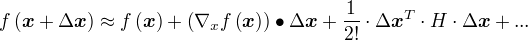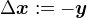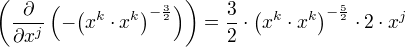The simplest Taylor approximation of a function f which has multiple parameters is:(where H is the Hesse Matrix)

In Electrodynamics, functions of the following form appear for the electrostatic potential:An example is:How do we calculate the value of this function?In order to find out, we develop the function into a Taylor Series with development point.We now actually plug the functionin:So, what is?Hence,Let. Then,So, what is?Hence,After plugging this into the original integral:With:Monopole momentumDipole momentumQuadrupole momentum